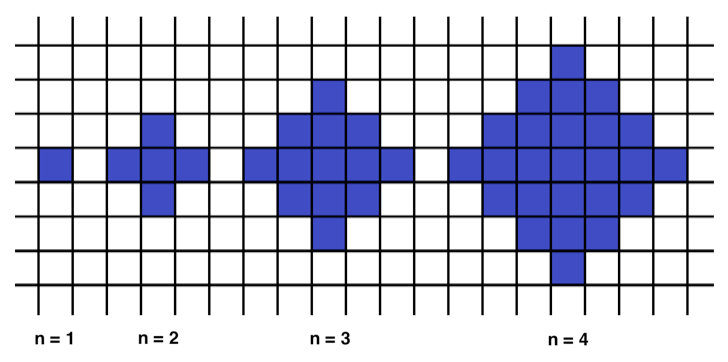# Space station🛰 to improve Proxonaut’s👩‍🚀 DSA

##### 🚀 Challenge 1 (Easy)

Write a function to check whether a given string is palindrome.

###### Example
• For `inputString = "aabaa"`, the output should be `solution(inputString) = true`;
• For `inputString = "abac"`, the output should be `solution(inputString) = false`;
• For `inputString = "a"`, the output should be `solution(inputString) = true`.
###### Solution
`Will be posted on 13th July`

##### 🚀 Challenge 2 (Mid-Easy)

Write a function to find the pair of adjacent elements that has the largest product from an array of integers and return that product.

###### Example
• For `inputArray = [3, 6, -2, -5, 7, 3]`, the output should be `solution(inputArray) = 21`.

`7` and `3` produce the largest product.

###### Solution
`Will be posted on 13th July`

##### 🚀 Challenge 3 (Moderate)

Below we will define an `n`-interesting polygon. Write a function to find the area of a polygon for a given `n`.

A `1`-interesting polygon is just a square with a side of length `1`. An `n`-interesting polygon is obtained by taking the `n - 1`-interesting polygon and appending `1`-interesting polygons to its rim, side by side. You can see the `1-, 2-, 3- and 4`-interesting polygons in the picture below.###### Example
• For `n = 2`, the output should be `solution(n) = 5`;
• For `n = 3`, the output should be `solution(n) = 13`.
###### Solution
`Will be posted on 13th July`

View Github